# Riesz system

(diff) ← Older revision | Latest revision (diff) | Newer revision → (diff)

A concept in the theory of orthogonal systems (cf. Orthonormal system). Let a complete system of functionsbe fixed in the space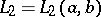. It is considered normalized, or, more generally, almost normalized, i.e. there are numbers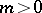and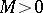for which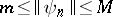for all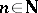. Weakening the requirement concerning the orthogonality of the system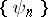one assumes that there exists a complete system of functions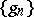in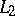such that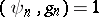,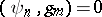for all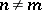. In particular, when the systemis orthonormal,for all. If a seriesconverges to a functionin, then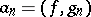for all. Thus it makes sense to call the numberthe-th Fourier coefficient of the functionwith respect to the system. In the proofs of a number of theorems in the theory of orthogonal series, the Bessel inequality and the Riesz–Fischer theorem are of great importance. In the general case these theorems are not valid, therefore one has to single out the special class of Riesz systems, i.e. systemssatisfying

1) for any functionthe series of the squares of the Fourier coefficients is absolutely convergent, i.e.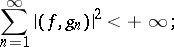2) for any sequence of numbers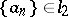there exists a functionfor which theare its Fourier coefficients with respect to the system, that is,for all.

The first requirement on the systemreplaces the Bessel inequality, the second the Riesz–Fischer theorem. N.K. Bari has proved (see ) that a systemis a Riesz system if and only if there exists a continuous linear operator, invertible in, such that the system of functions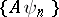is complete and orthonormal. Therefore, a Riesz system is also called a Riesz basis, equivalent to an orthonormal basis. Bari has indicated a convenient criterion for being a Riesz system. A complete system of functionsinis a Riesz system if and only if the Gram matrixdetermines a continuous invertible linear operator in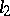. Under an arbitrary permutation of the elements of a Riesz system one obtains again a Riesz system. Conversely, if a basis inis still a basis after any permutation of its elements, then by normalizing it one obtains a Riesz system. A natural generalization of a Riesz system is obtained by replacingby the closure of the linear span of a systemwith respect to the norm of the Hilbert space from which the elementsare taken (see ).

How to Cite This Entry:
Riesz system. Encyclopedia of Mathematics. URL: http://encyclopediaofmath.org/index.php?title=Riesz_system&oldid=16931
This article was adapted from an original article by V.F. Emel'yanov (originator), which appeared in Encyclopedia of Mathematics - ISBN 1402006098. See original article